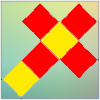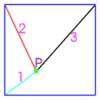Search by Topic

Resources tagged with Pythagoras' theorem similar to Small Steps:

Filter by: Content type:
Age range:
Challenge level:

There are 79 results

Broad Topics > Pythagoras and Trigonometry > Pythagoras' theoremGolden Construction

Age 16 to 18 Challenge Level:

Draw a square and an arc of a circle and construct the Golden rectangle. Find the value of the Golden Ratio.Far Horizon

Age 14 to 16 Challenge Level:

An observer is on top of a lighthouse. How far from the foot of the lighthouse is the horizon that the observer can see?Logosquares

Age 16 to 18 Challenge Level:

Ten squares form regular rings either with adjacent or opposite vertices touching. Calculate the inner and outer radii of the rings that surround the squares.Holly

Age 14 to 16 Challenge Level:

The ten arcs forming the edges of the "holly leaf" are all arcs of circles of radius 1 cm. Find the length of the perimeter of the holly leaf and the area of its surface.Under the Ribbon

Age 14 to 16 Challenge Level:

A ribbon is nailed down with a small amount of slack. What is the largest cube that can pass under the ribbon ?Retracircles

Age 16 to 18 Challenge Level:

Four circles all touch each other and a circumscribing circle. Find the ratios of the radii and prove that joining 3 centres gives a 3-4-5 triangle.The Dodecahedron

Age 16 to 18 Challenge Level:

What are the shortest distances between the centres of opposite faces of a regular solid dodecahedron on the surface and through the middle of the dodecahedron?Pythagoras for a Tetrahedron

Age 16 to 18 Challenge Level:

In a right-angled tetrahedron prove that the sum of the squares of the areas of the 3 faces in mutually perpendicular planes equals the square of the area of the sloping face. A generalisation. . . .Squ-areas

Age 14 to 16 Challenge Level:

Three squares are drawn on the sides of a triangle ABC. Their areas are respectively 18 000, 20 000 and 26 000 square centimetres. If the outer vertices of the squares are joined, three more. . . .Belt

Age 16 to 18 Challenge Level:

A belt of thin wire, length L, binds together two cylindrical welding rods, whose radii are R and r, by passing all the way around them both. Find L in terms of R and r.Compare Areas

Age 14 to 16 Challenge Level:

Which has the greatest area, a circle or a square inscribed in an isosceles, right angle triangle?Baby Circle

Age 16 to 18 Challenge Level:

A small circle fits between two touching circles so that all three circles touch each other and have a common tangent? What is the exact radius of the smallest circle?Star Gazing

Age 14 to 16 Challenge Level:

Find the ratio of the outer shaded area to the inner area for a six pointed star and an eight pointed star.Age 14 to 16 Challenge Level:

A 1 metre cube has one face on the ground and one face against a wall. A 4 metre ladder leans against the wall and just touches the cube. How high is the top of the ladder above the ground?Strange Rectangle

Age 16 to 18 Challenge Level:

ABCD is a rectangle and P, Q, R and S are moveable points on the edges dividing the edges in certain ratios. Strangely PQRS is always a cyclic quadrilateral and you can find the angles.Get Cross

Age 14 to 16 Challenge Level:

A white cross is placed symmetrically in a red disc with the central square of side length sqrt 2 and the arms of the cross of length 1 unit. What is the area of the disc still showing?Are You Kidding

Age 14 to 16 Challenge Level:

If the altitude of an isosceles triangle is 8 units and the perimeter of the triangle is 32 units.... What is the area of the triangle?Circle Packing

Age 14 to 16 Challenge Level:

Equal circles can be arranged so that each circle touches four or six others. What percentage of the plane is covered by circles in each packing pattern? ...Two Circles

Age 14 to 16 Challenge Level:

Draw two circles, each of radius 1 unit, so that each circle goes through the centre of the other one. What is the area of the overlap?Crescents and Triangles

Age 14 to 16 Challenge Level:

Can you find a relationship between the area of the crescents and the area of the triangle?Grid Lockout

Age 14 to 16 Challenge Level:

What remainders do you get when square numbers are divided by 4?Circle Scaling

Age 14 to 16 Challenge Level:

Describe how to construct three circles which have areas in the ratio 1:2:3.Inscribed in a Circle

Age 14 to 16 Challenge Level:

The area of a square inscribed in a circle with a unit radius is, satisfyingly, 2. What is the area of a regular hexagon inscribed in a circle with a unit radius?Classic Cube

Age 16 to 18 Challenge Level:

The net of a cube is to be cut from a sheet of card 100 cm square. What is the maximum volume cube that can be made from a single piece of card?Incircles Explained

Age 16 to 18The Fire-fighter's Car Keys

Age 14 to 16 Challenge Level:

A fire-fighter needs to fill a bucket of water from the river and take it to a fire. What is the best point on the river bank for the fire-fighter to fill the bucket ?.Partly Circles

Age 14 to 16 Challenge Level:

What is the same and what is different about these circle questions? What connections can you make?Six Discs

Age 14 to 16 Challenge Level:

Six circular discs are packed in different-shaped boxes so that the discs touch their neighbours and the sides of the box. Can you put the boxes in order according to the areas of their bases?Spherical Triangles on Very Big Spheres

Age 16 to 18 Challenge Level:

Shows that Pythagoras for Spherical Triangles reduces to Pythagoras's Theorem in the plane when the triangles are small relative to the radius of the sphere.Semi-square

Age 14 to 16 Challenge Level:

What is the ratio of the area of a square inscribed in a semicircle to the area of the square inscribed in the entire circle?Some(?) of the Parts

Age 14 to 16 Challenge Level:

A circle touches the lines OA, OB and AB where OA and OB are perpendicular. Show that the diameter of the circle is equal to the perimeter of the triangle30-60-90 Polypuzzle

Age 16 to 18 Challenge Level:

Re-arrange the pieces of the puzzle to form a rectangle and then to form an equilateral triangle. Calculate the angles and lengths.At a Glance

Age 14 to 16 Challenge Level:

The area of a regular pentagon looks about twice as a big as the pentangle star drawn within it. Is it?Where Is the Dot?

Age 14 to 16 Challenge Level:

A dot starts at the point (1,0) and turns anticlockwise. Can you estimate the height of the dot after it has turned through 45 degrees? Can you calculate its height?Reach for Polydron

Age 16 to 18 Challenge Level:

A tetrahedron has two identical equilateral triangles faces, of side length 1 unit. The other two faces are right angled isosceles triangles. Find the exact volume of the tetrahedron.Ball Packing

Age 14 to 16 Challenge Level:

If a ball is rolled into the corner of a room how far is its centre from the corner?Orthogonal Circle

Age 16 to 18 Challenge Level:

Given any three non intersecting circles in the plane find another circle or straight line which cuts all three circles orthogonally.Rectangular Pyramids

Age 14 to 18 Challenge Level:

Is the sum of the squares of two opposite sloping edges of a rectangular based pyramid equal to the sum of the squares of the other two sloping edges?Medallions

Age 14 to 16 Challenge Level:

Three circular medallions fit in a rectangular box. Can you find the radius of the largest one?Three Four Five

Age 14 to 16 Challenge Level:

Two semi-circles (each of radius 1/2) touch each other, and a semi-circle of radius 1 touches both of them. Find the radius of the circle which touches all three semi-circles.Napkin

Age 14 to 16 Challenge Level:

A napkin is folded so that a corner coincides with the midpoint of an opposite edge . Investigate the three triangles formed .Pythagoras Mod 5

Age 16 to 18 Challenge Level:

Prove that for every right angled triangle which has sides with integer lengths: (1) the area of the triangle is even and (2) the length of one of the sides is divisible by 5.Age 14 to 16 Challenge Level:

The sides of a triangle are 25, 39 and 40 units of length. Find the diameter of the circumscribed circle.Take a Square

Age 14 to 16 Challenge Level:

Cut off three right angled isosceles triangles to produce a pentagon. With two lines, cut the pentagon into three parts which can be rearranged into another square.Semi-detached

Age 14 to 16 Challenge Level:

A square of area 40 square cms is inscribed in a semicircle. Find the area of the square that could be inscribed in a circle of the same radius.Square World

Age 16 to 18 Challenge Level:

P is a point inside a square ABCD such that PA= 1, PB = 2 and PC = 3. How big is angle APB ?Where to Land

Age 14 to 16 Challenge Level:

Chris is enjoying a swim but needs to get back for lunch. If she can swim at 3 m/s and run at 7m/sec, how far along the bank should she land in order to get back as quickly as possible?Xtra

Age 14 to 18 Challenge Level:

Find the sides of an equilateral triangle ABC where a trapezium BCPQ is drawn with BP=CQ=2 , PQ=1 and AP+AQ=sqrt7 . Note: there are 2 possible interpretations.Slippage

Age 14 to 16 Challenge Level:

A ladder 3m long rests against a wall with one end a short distance from its base. Between the wall and the base of a ladder is a garden storage box 1m tall and 1m high. What is the maximum distance. . . .Rhombus in Rectangle

Age 14 to 16 Challenge Level:

Take any rectangle ABCD such that AB > BC. The point P is on AB and Q is on CD. Show that there is exactly one position of P and Q such that APCQ is a rhombus.